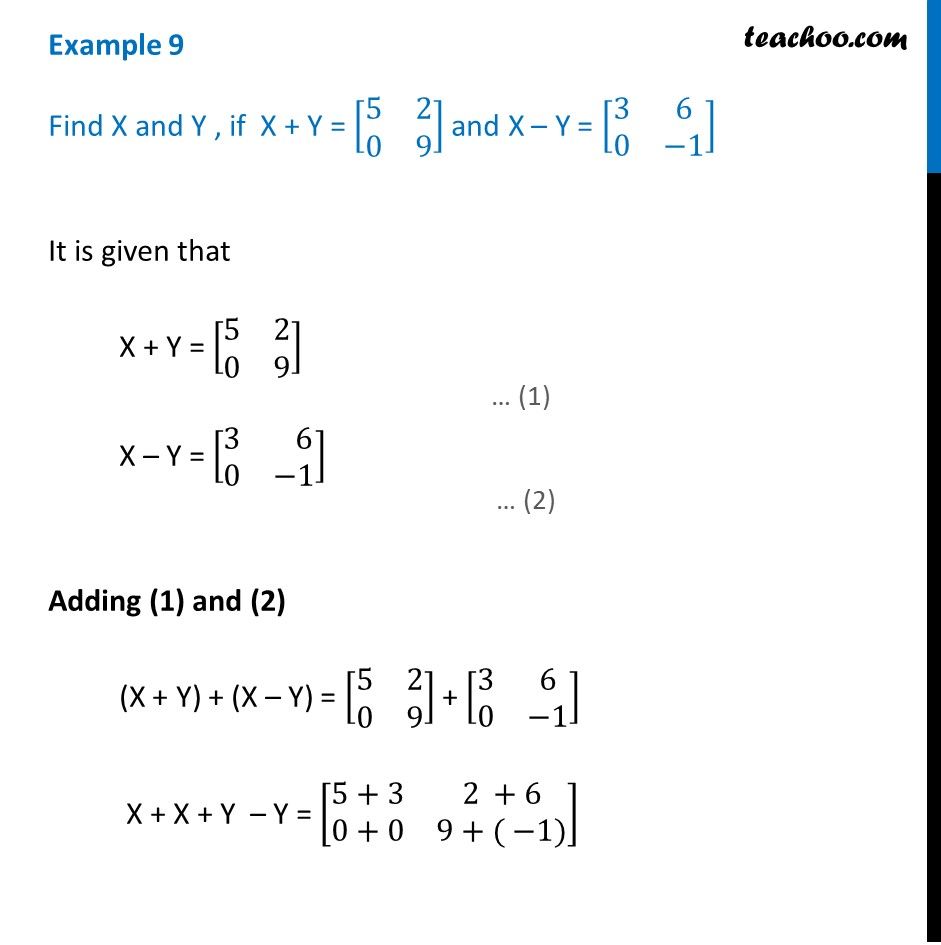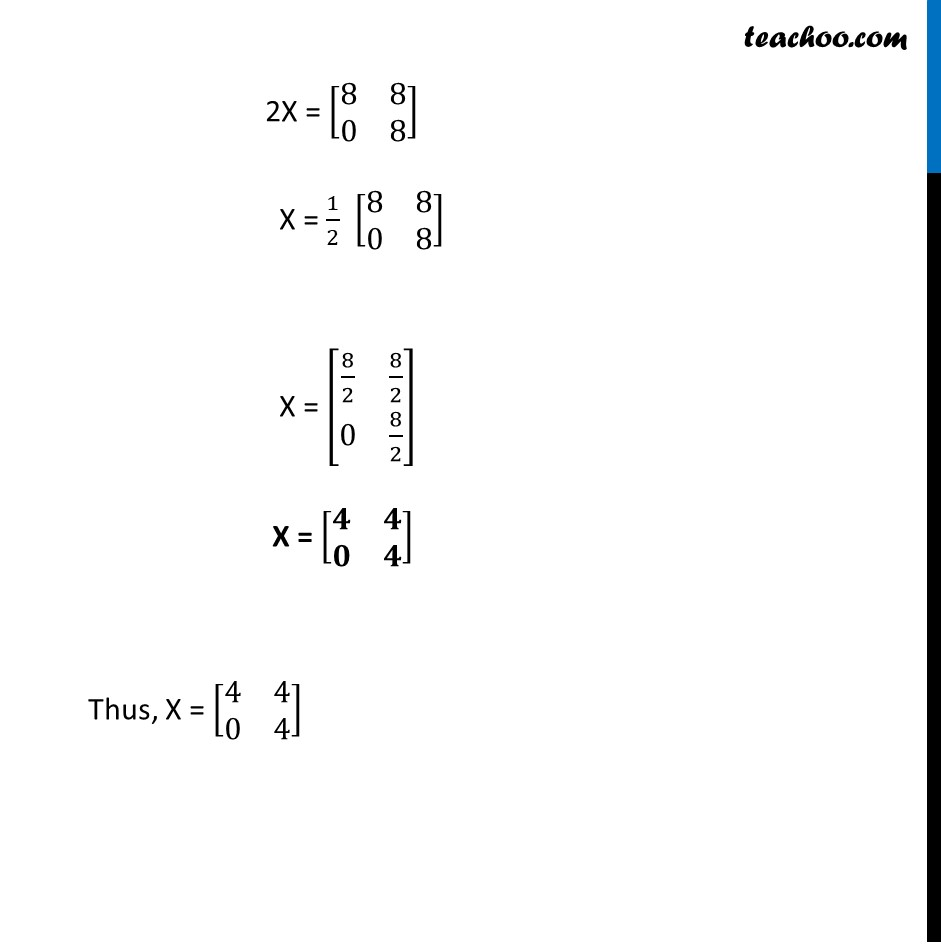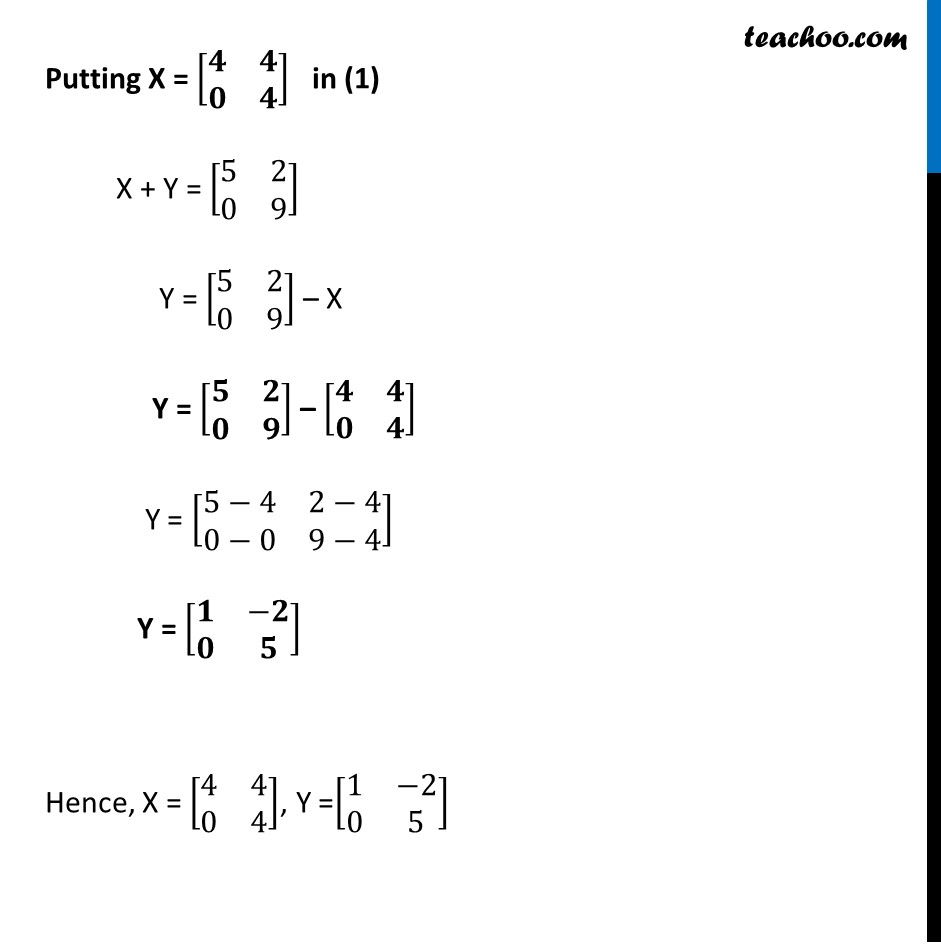Chapter 3 Class 12 Matrices
Concept wiseLearn in your speed, with individual attention - Teachoo Maths 1-on-1 Class

### Transcript

Example 9 Find X and Y , if X + Y = [■8(5&2@0&9)] and X – Y = [■8(3&6@0&−1)] It is given that X + Y = [■8(5&2@0&9)] X – Y = [■8(3& 6@0&−1)] Adding (1) and (2) (X + Y) + (X – Y) = [■8(5&2@0&9)] + [■8(3&6@0&−1)] X + X + Y – Y = [■8(5+3&2 +6@0+0&9+( −1))] 2X = [■8(8&8@0&8)] X = 1/2 [■8(8&8@0&8)] X = [■8(8/2&8/2@0&8/2)] X = [■8(𝟒&𝟒@𝟎&𝟒)] Thus, X = [■8(4&4@0&4)] Putting X = [■8(𝟒&𝟒@𝟎&𝟒)] in (1) X + Y = [■8(5&2@0&9)] Y = [■8(5&2@0&9)] – X Y = [■8(𝟓&𝟐@𝟎&𝟗)] – [■8(𝟒&𝟒@𝟎&𝟒)] Y = [■8(5−4&2−4@0−0&9−4)] Y = [■8(𝟏&−𝟐@𝟎&𝟓)] Hence, X = [■8(4&4@0&4)], Y =[■8(1&−2@0&5)]